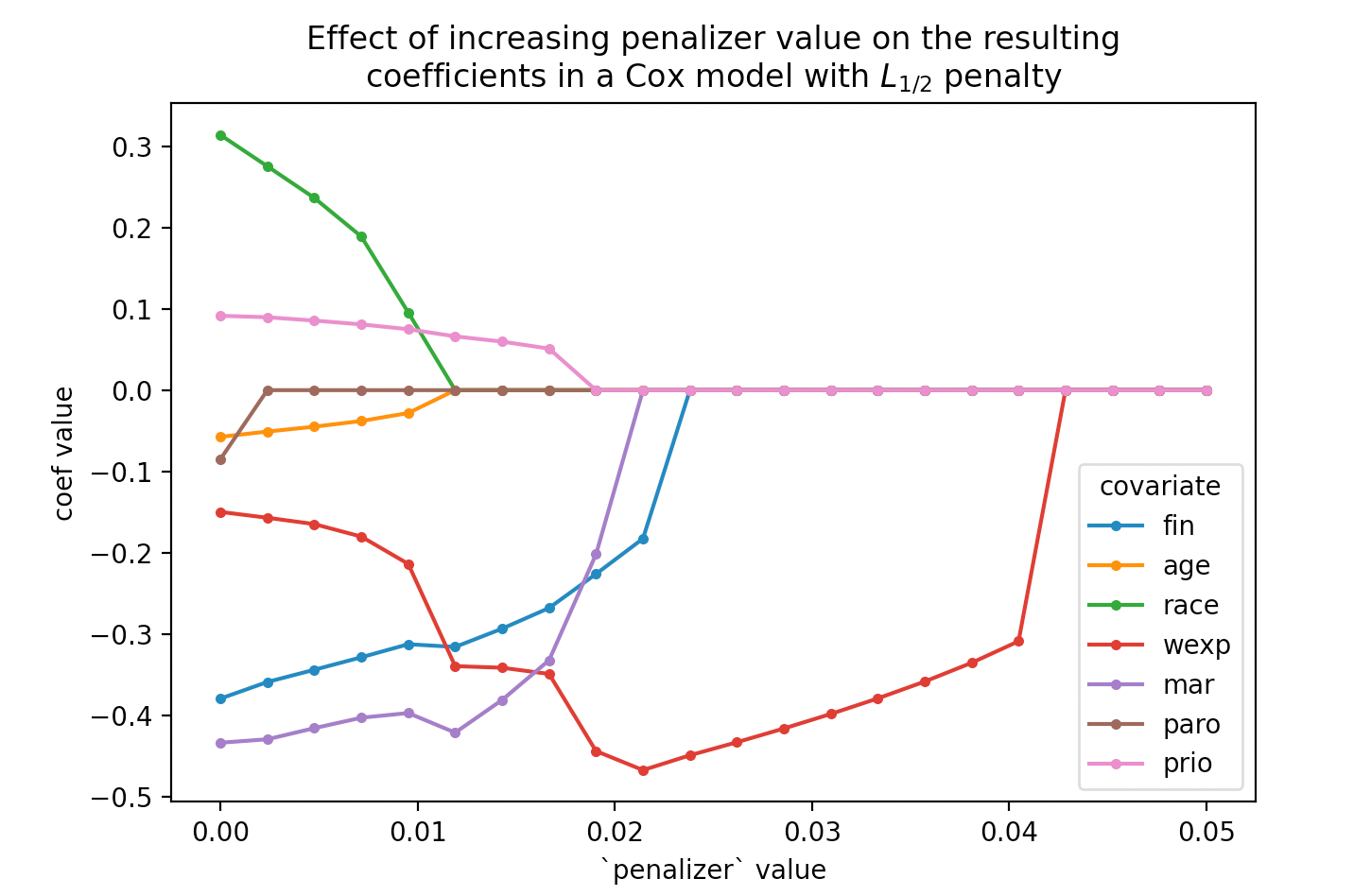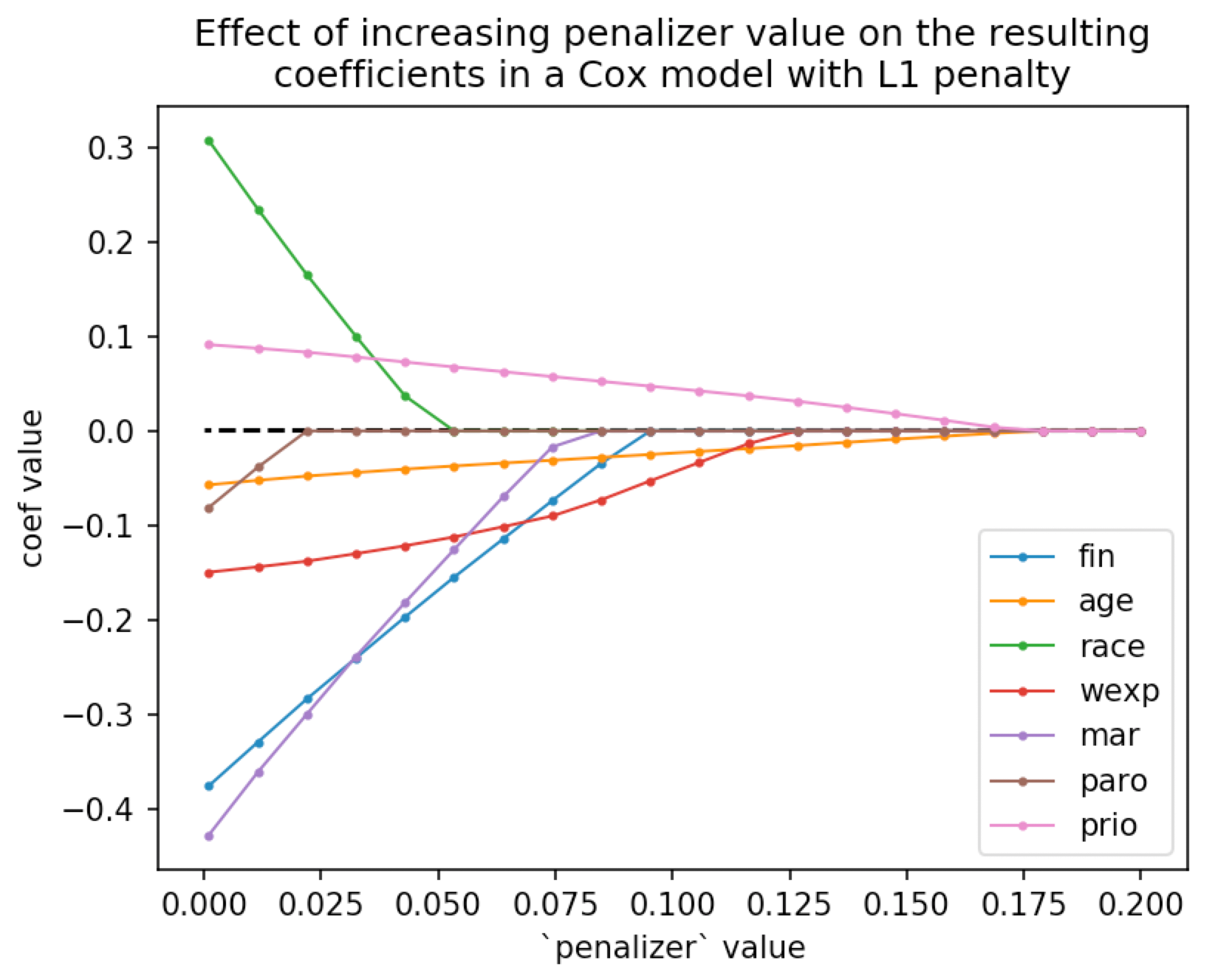# An L½ penalty in Cox Regression

Posted by Cameron Davidson-Pilon on

Following up from a previous blog post where we explored how to implement an $$L_1$$ and elastic net penalty to induce sparsity, a paper, by Xu Z B, Zhang H, Wang Y, et al., explores what a $$L_{1/2}$$ penalty is and how to implement it.

But first, I think we are familiar with an $$L_1$$ penalty, but what is an $$L_0$$ penalty then? If you work out the math, it is a penalty that counts the number of non-zero coefficients, independent of the magnitude of the coefficients:

$$ll^*(\theta, x) = \sum_i^N ll(\theta, x_i) - \lambda \sum_{k=0}^D 1_{\theta_k \ne 0}$$

where $$D$$ is the number of potential parameters. Thinking about this for a moment, this means that the $$L_0$$ penalty minimizes the AIC, since the AIC is:

$$AIC = -2 ll + 2D^*$$

where $$D^*$$ is the number of parameters in the model. It turns out that $$L_0$$ penalties encourage lots of sparsity in their solutions, much more than $$L_1$$.

Given that, the $$L_{1/2}$$ penalty is the balance between penalizing the magnitudes of the coefficients and encouraging lots of sparsity. The paper linked above gives reasons why $$L_{1/2}$$ is perhaps superior to both $$L_1$$ and $$L_0$$. Importantly, solving $$L_0$$ is NP-hard because it involves a combinatorial explosion of potential solutions that can't be solved with gradient methods.

The authors provide a very simple algorithm for solving the $$L_{1/2}$$ problem, see Section 3 of the paper. It involves repeatedly solving a related $$L_1$$ problem with updating coefficient-specific penalizer values. In lifelines, we recently introduced the ability to set specific coefficient penalizer values, and we can solve $$L_1$$ problems too. Let's see if we can solve $$L_{1/2}$$ problems now:

def l_one_half_cox(lambda_, df, T, E):
EPSILON = 0.00001

weights = lambda_ * np.ones(df.shape-2)
cph = CoxPHFitter(l1_ratio=1.0, penalizer=weights)
cph.fit(rossi, "week", "arrest")
max_iter = 20
i = 1

while i < max_iter:
weights = lambda_ / (np.sqrt((cph.params_.abs()).values) + EPSILON)
cph = CoxPHFitter(l1_ratio=1.0, penalizer=weights)
cph.fit(rossi, "week", "arrest")
i += 1

return cph.params_


In the above code, we repeatedly solve a new $$L_1$$ problem with updated penalizer weights. This, according to the authors and my own assumption that it can be extended easily to the Cox model, gives us our $$L_{1/2}$$ solution. Graphically, we can vary the lambda_ parameter can see how the coefficient solutions change:Compare this to our $$L_1$$ solution: